Get instant live expert help with Excel or Google Sheets“My Excelchat expert helped me in less than 20 minutes, saving me what would have been 5 hours of work!”

#### Post your problem and you’ll get Expert help in seconds.

Your message must be at least 40 characters
Our professional Expert are available now. Your privacy is guaranteed.

# Count visible rows only with criteria

While working with Excel, we are able to perform calculations that consider only visible rows by using key functions such as SUMPRODUCT, SUBTOTAL, OFFSET, MIN and ROW.  This step by step tutorial will assist all levels of Excel users in counting only visible rows with criteria.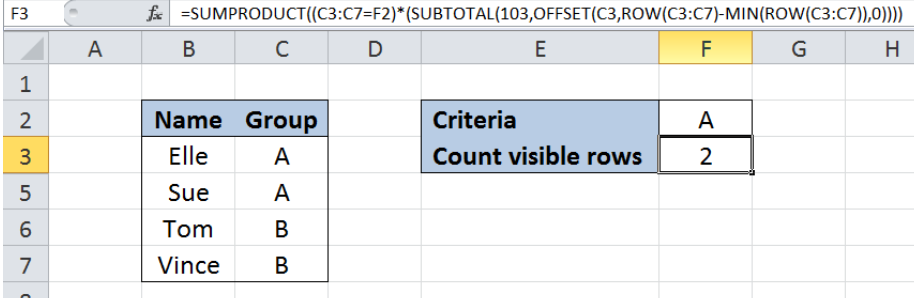Figure 1. Final result: Count visible rows only with criteria

Final formula:` =SUMPRODUCT((C3:C7=F2)*(SUBTOTAL(103,OFFSET(C3,ROW(C3:C7)-MIN(ROW(C3:C7)),0))))`

## Syntax of SUMPRODUCT Function

SUMPRODUCT returns the sum of the products of two or more components in the given arrays

`=SUMPRODUCT(array1, [array2], [array3], ...)`

• array – a range of values or cells whose values we want to multiply with other values and then add
• All array arguments must be in the same size
• Non-numeric values in an array are treated as zeros

## Syntax of SUBTOTAL Function

SUBTOTAL returns a subtotal in the list or data

`=SUBTOTAL(function_num,ref1,[ref2],...])`

• function_num   – a number specifying the function to use in calculating the subtotal; 1 to 11 (includes hidden values) while 101 to 111 (ignores hidden values); see below table for the list of functions and function numbers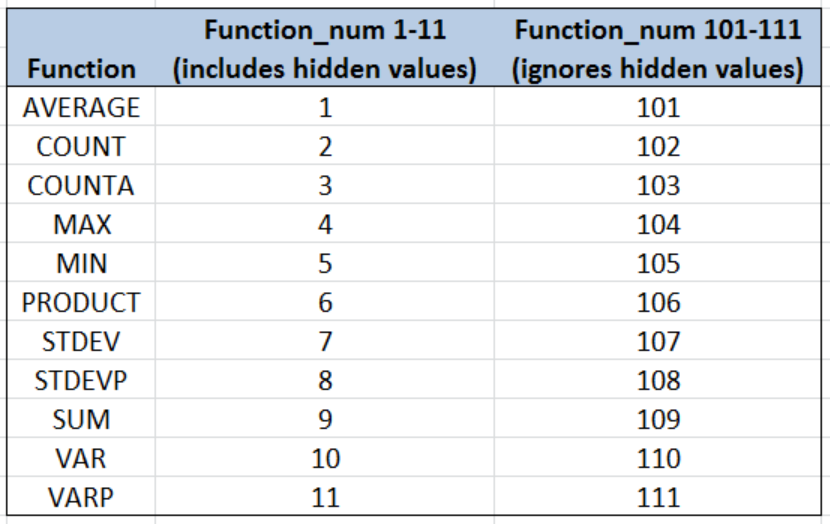Figure 2.  SUBTOTAL functions and corresponding function_num values

• ref1   – the first range or reference whose subtotal we want to find
• Only ref1 is required; succeeding references are optional. Named ranges or references 2 to 254 for which you want the subtotal

## Syntax of OFFSET function

OFFSET returns a single cell or range of cells based from the specified number of rows and columns from a reference

`=OFFSET(reference, rows, cols, [height], [width])`

• reference  – the reference where we base the offset; must be a cell or range of adjacent cells
• rows  – number of rows up or down the reference that specifies the cell we are referring to; when row value is positive, it refers to rows below the starting reference; otherwise, it refers to above the reference
• cols  – number of columns to the left (negative value) or right (positive value) of the reference that specifies the cell we are referring to
• height  Optional; the number of rows that we want the returned reference to be; height value must be positive
• width Optional; the number of columns that we want the returned reference to be; width value must be positive
• If height or width is omitted,default is the same height or width as the reference
• OFFSET doesn’t move any cells but only returns a reference

## Syntax of MIN function

MIN function returns the lowest value in a data set

`=MIN(number1, [number2], ...)  `

• number1, number2, … – the numbers for which we want to find the lowest value; only number1 is required; succeeding numbers are optional
• The arguments could be numbers, array or reference to cells containing numbers

## Syntax of the ROW function

ROW function returns the row number of a reference

`=ROW(reference)`

• reference – The cell or range of cells whose row number we want to determine

## Setting up Our Data

Our table consists of two columns: Name (column B) and Group (column C). In cell F2, we enter our criteria which is Group “A”.  We want to count the visible rows for Group A and record the result in F3.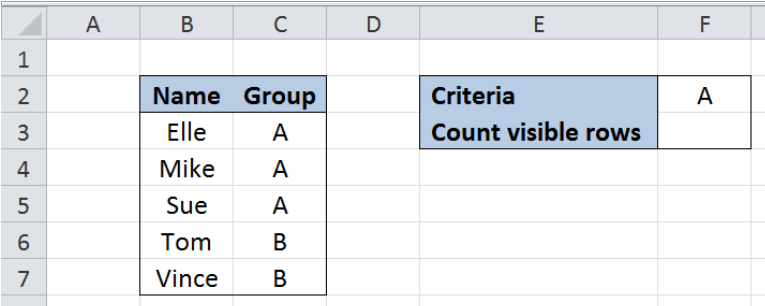Figure 3. Sample data to count visible rows only with criteria

## Count visible rows only for Group A

In order to count visible rows only for a given criteria, we will be using a formula with SUMPRODUCT, SUBTOTAL, OFFSET, ROW and MIN functions.  Let us follow these steps:

Step 1.  Select cell F3

Step 2.  Enter the formula: `=SUMPRODUCT((C3:C7=F2)*(SUBTOTAL(103,OFFSET(C3,ROW(C3:C7)-MIN(ROW(C3:C7)),0))))`

Step 3:  Press ENTER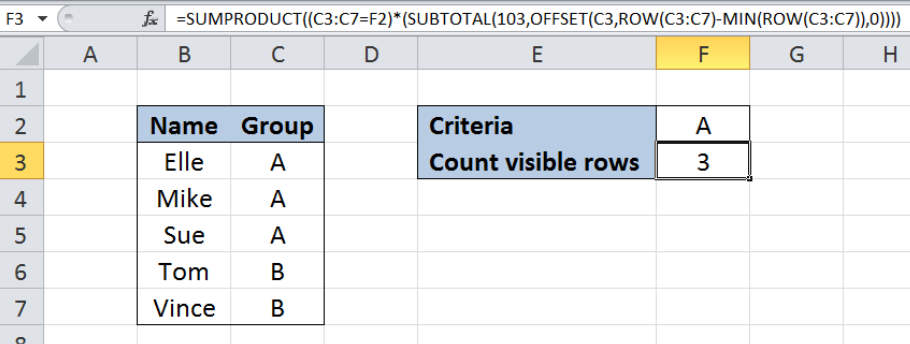Figure 4. Entering the formula to count visible rows only for Group A

With all rows displayed, the resulting count in F3 is 3, which corresponds to the three rows with  Group A in rows 3,4 and 5.

Now let us hide row 4 and count the visible rows for Group A.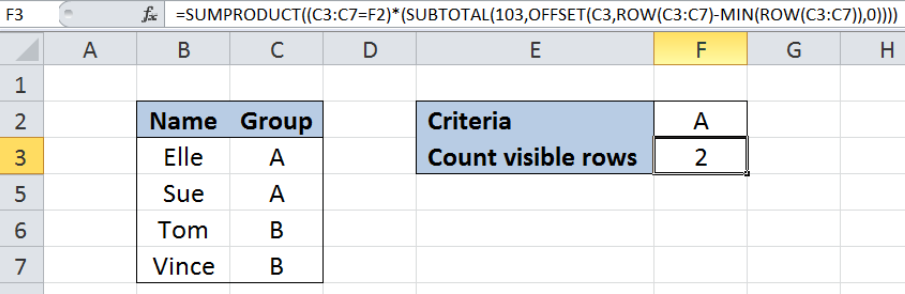Figure 5. Counting only visible rows for Group A, excluding row 4

With row 4 hidden, our formula cleverly counts only the visible rows for Group A, rows 3 and 5.

## How the formula works

`=SUMPRODUCT((C3:C7=F2)*(SUBTOTAL(103,OFFSET(C3,ROW(C3:C7)-MIN(ROW(C3:C7)),0))))  `

The main function here is the SUMPRODUCT, which has two criteria:

• `(C3:C7=F2)` evaluates that the value in column C is equal to our criteria in F2, Group “A

It results to an array where the value of TRUE is 1, while FALSE is 0.  The resulting arrayis `{1;1;1;0;0}`

• `(SUBTOTAL(103,OFFSET(C3,ROW(C3:C7)-MIN(ROW(C3:C7)),0)))` counts only the visible or non-hidden rows

From Figure 2 above, function_num 103 for SUBTOTAL means it counts only non-hidden or visible cells.

For the OFFSET function, the number of columns is 0 so it refers to the current column of C3 while the number of rows is an array from `ROW(C3:C7)-MIN(ROW(C3:C7))`

ROW returns the row number while MIN returns the lowest value in a set of data.  This part of the formula results to `{3;4;5;6;7}-MIN({3;4;5;6;7}) = {3;4;5;6;7} - 3 = {0;1;2;3;4}`

With  {0;1;2;3;4} as the rows argument for OFFSET, the resulting array is `{C3;C4;C5;C6;C7}` or `{A;A;A;B;B}`.  When fed to the SUBTOTAL where hidden values such as C4 are not counted, the resulting array becomes `{1;0;1;1;1}`

Finally, the SUMPRODUCT multiplies or combines the two arrays `{1;1;1;0;0}* {1;0;1;1;1}` and results to `{1;0;1;0;0}`, the sum of which is 2.

As a result, the value in F3 is 2, which corresponds to the two visible rows that satisfy our criteria, Group “A”.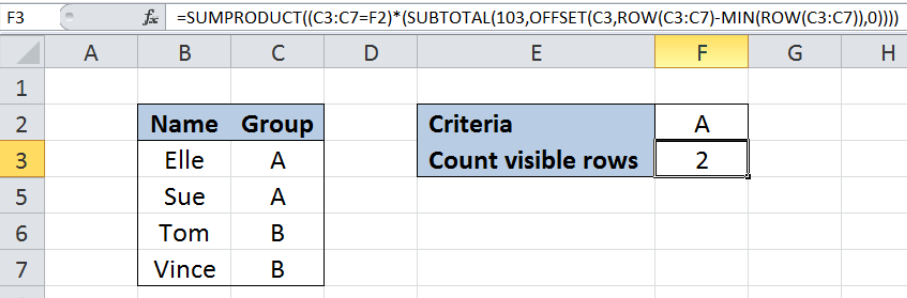Figure 6.  Output: Count visible rows only for Group A

Most of the time, the problem you will need to solve will be more complex than a simple application of a formula or function. If you want to save hours of research and frustration, try our live Excelchat service! Our Excel Experts are available 24/7 to answer any Excel question you may have. We guarantee a connection within 30 seconds and a customized solution within 20 minutes.

### Did this post not answer your question? Get a solution from connecting with the expert.Another blog reader asked this question today on Excelchat:
Solution examplesHi, I need a formula to work out how many values are less than a certain time value, simple "countif" do not work as it is a filtered spreadsheet. Here's the formula that I have got so far: SUMPRODUCT(SUBTOTAL(3,OFFSET(Current_Month!N\$2:N\$10000,ROW(Current_Month!N\$2:N\$10000)-MIN(ROW(Current_Month!N\$2:N\$10000)),,1)),--(Current_Month!N\$2:N\$10000>"24:0:0")
Solved by K. L. in 30 minsUse the Vlookup Function to complete the "employee" column of table 2. Use "job Id" from table 2 as your lookup_value(s) and table 1 as your reference.
Solved by C. H. in 16 minsIf a cell in another sheet is populated I need a vlookup done. If the cell is not populated I need the cell to return blank.
Solved by T. D. in 60 minsI am trying to make a chart that turns a week range red if nothing is entered in the range. If something is entered then I would like it to turn green. Please Help
Solved by E. U. in 43 minsI need a check box to show/hide an answer of an if function
Solved by Z. U. in 23 mins## Subscribe to Excelchat.coAnother blog reader asked this question today on Excelchat: